# 33 DC Voltmeters and Ammeters

### Learning Objectives

By the end of this section, you will be able to:

• Explain why a voltmeter must be connected in parallel with the circuit.
• Draw a diagram showing an ammeter correctly connected in a circuit.
• Describe how a galvanometer can be used as either a voltmeter or an ammeter.
• Find the resistance that must be placed in series with a galvanometer to allow it to be used as a voltmeter with a given reading.
• Explain why measuring the voltage or current in a circuit can never be exact.

Voltmeters measure voltage, whereas ammeters measure current. Some of the meters in automobile dashboards, digital cameras, cell phones, and tuner-amplifiers are voltmeters or ammeters. (See Figure 1.) The internal construction of the simplest of these meters and how they are connected to the system they monitor give further insight into applications of series and parallel connections.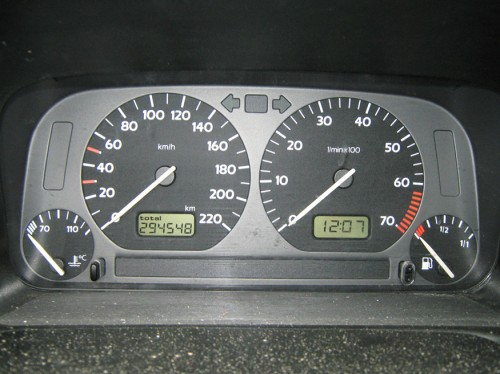Figure 1. The fuel and temperature gauges (far right and far left, respectively) in this 1996 Volkswagen are voltmeters that register the voltage output of “sender” units, which are hopefully proportional to the amount of gasoline in the tank and the engine temperature. (credit: Christian Giersing)

Voltmeters are connected in parallel with whatever device’s voltage is to be measured. A parallel connection is used because objects in parallel experience the same potential difference. (See Figure 2, where the voltmeter is represented by the symbol V.) Ammeters are connected in series with whatever device’s current is to be measured. A series connection is used because objects in series have the same current passing through them. (See Figure 3, where the ammeter is represented by the symbol A.)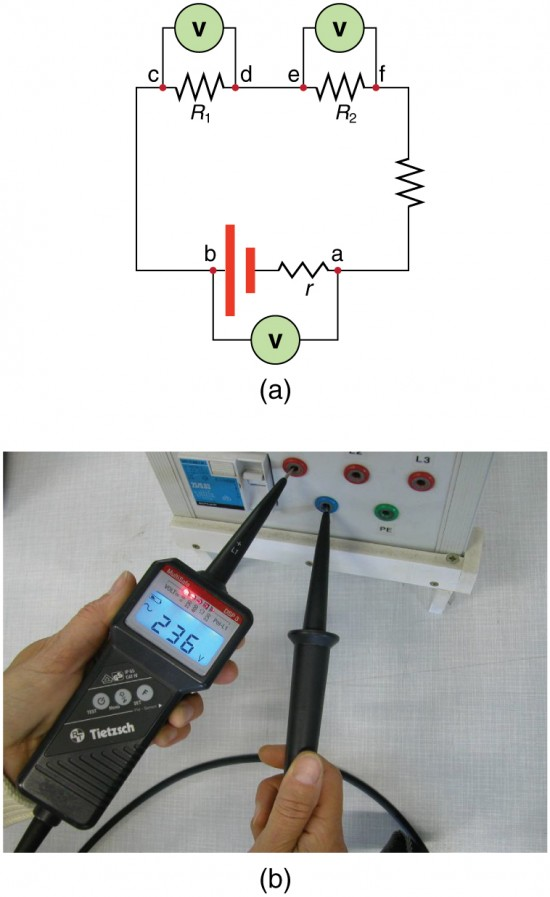Figure 2. (a) To measure potential differences in this series circuit, the voltmeter (V) is placed in parallel with the voltage source or either of the resistors. Note that terminal voltage is measured between points a and b. It is not possible to connect the voltmeter directly across the emf without including its internal resistance, r. (b) A digital voltmeter in use. (credit: Messtechniker, Wikimedia Commons)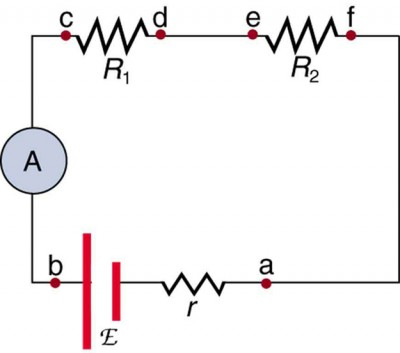Figure 3. An ammeter (A) is placed in series to measure current. All of the current in this circuit flows through the meter. The ammeter would have the same reading if located between points d and e or between points f and a as it does in the position shown. (Note that the script capital E stands for emf, and r stands for the internal resistance of the source of potential difference.)

### Analog Meters: Galvanometers

Analog meters have a needle that swivels to point at numbers on a scale, as opposed to digital meters, which have numerical readouts similar to a hand-held calculator. The heart of most analog meters is a device called a galvanometer, denoted by G. Current flow through a galvanometer, IG, produces a proportional needle deflection. (This deflection is due to the force of a magnetic field upon a current-carrying wire.)

The two crucial characteristics of a given galvanometer are its resistance and current sensitivity. Current sensitivity is the current that gives a full-scale deflection of the galvanometer’s needle, the maximum current that the instrument can measure. For example, a galvanometer with a current sensitivity of 50 μA has a maximum deflection of its needle when 50 μA flows through it, reads half-scale when 25 μA flows through it, and so on. If such a galvanometer has a 25-Ω resistance, then a voltage of only V = IR = (50 μA)(25 Ω) = 1.25 mV produces a full-scale reading. By connecting resistors to this galvanometer in different ways, you can use it as either a voltmeter or ammeter that can measure a broad range of voltages or currents.

### Galvanometer as Voltmeter

Figure 4 shows how a galvanometer can be used as a voltmeter by connecting it in series with a large resistance, R. The value of the resistance R is determined by the maximum voltage to be measured. Suppose you want 10 V to produce a full-scale deflection of a voltmeter containing a 25-Ω galvanometer with a 50-μA sensitivity. Then 10 V applied to the meter must produce a current of 50 μA. The total resistance must be

${R}_{\text{tot}}=R+r=\frac{V}{I}=\frac{10\text{ V}}{50\text{ }\mu \text{A}}=200\text{ k}\Omega\\$ or
$R={R}_{\text{tot}}-r=200\text{ k}\Omega-25\text{ }\Omega \approx 200\text{ k}\Omega \\$.

(R is so large that the galvanometer resistance, r, is nearly negligible.) Note that 5 V applied to this voltmeter produces a half-scale deflection by producing a 25-μA current through the meter, and so the voltmeter’s reading is proportional to voltage as desired. This voltmeter would not be useful for voltages less than about half a volt, because the meter deflection would be small and difficult to read accurately. For other voltage ranges, other resistances are placed in series with the galvanometer. Many meters have a choice of scales. That choice involves switching an appropriate resistance into series with the galvanometer.Figure 4. A large resistance R placed in series with a galvanometer G produces a voltmeter, the full-scale deflection of which depends on the choice of R. The larger the voltage to be measured, the larger R must be. (Note that r represents the internal resistance of the galvanometer.)

### Galvanometer as Ammeter

The same galvanometer can also be made into an ammeter by placing it in parallel with a small resistance R, often called the shunt resistance, as shown in Figure 5. Since the shunt resistance is small, most of the current passes through it, allowing an ammeter to measure currents much greater than those producing a full-scale deflection of the galvanometer. Suppose, for example, an ammeter is needed that gives a full-scale deflection for 1.0 A, and contains the same 25-Ω galvanometer with its 50-μA sensitivity. Since R and r are in parallel, the voltage across them is the same. These IR drops are IR = IGr so that $IR=\frac{{I}_{\text{G}}}{I}=\frac{R}{r}\\$. Solving for R, and noting that IG is 50 μA and I is 0.999950 A, we have

$R=r\frac{{I}_{\text{G}}}{I}=\left(25\text{ }\Omega\right)\frac{50\text{ }\mu\text{A}}{0.999950\text{ A}}=1.25\times 10^{-3}\text{ }\Omega\\$.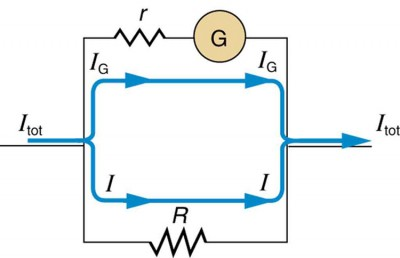Figure 5. A small shunt resistance R placed in parallel with a galvanometer G produces an ammeter, the full-scale deflection of which depends on the choice of R. The larger the current to be measured, the smaller R must be. Most of the current (I) flowing through the meter is shunted through R to protect the galvanometer. (Note that r represents the internal resistance of the galvanometer.) Ammeters may also have multiple scales for greater flexibility in application. The various scales are achieved by switching various shunt resistances in parallel with the galvanometer—the greater the maximum current to be measured, the smaller the shunt resistance must be.

## Taking Measurements Alters the Circuit

When you use a voltmeter or ammeter, you are connecting another resistor to an existing circuit and, thus, altering the circuit. Ideally, voltmeters and ammeters do not appreciably affect the circuit, but it is instructive to examine the circumstances under which they do or do not interfere. First, consider the voltmeter, which is always placed in parallel with the device being measured. Very little current flows through the voltmeter if its resistance is a few orders of magnitude greater than the device, and so the circuit is not appreciably affected. (See Figure 6(a).) (A large resistance in parallel with a small one has a combined resistance essentially equal to the small one.) If, however, the voltmeter’s resistance is comparable to that of the device being measured, then the two in parallel have a smaller resistance, appreciably affecting the circuit. (See Figure 6(b).) The voltage across the device is not the same as when the voltmeter is out of the circuit.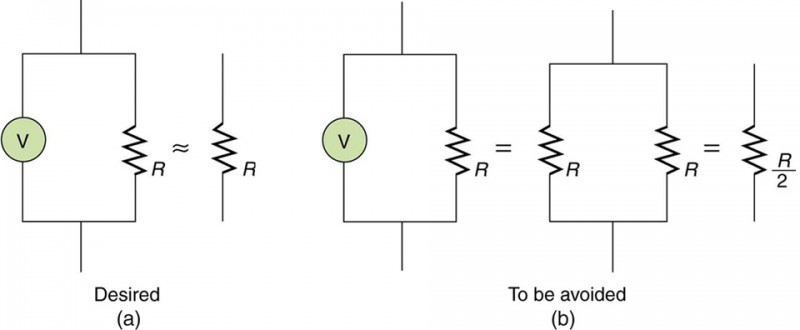Figure 6. (a) A voltmeter having a resistance much larger than the device (RVoltmeter>>R) with which it is in parallel produces a parallel resistance essentially the same as the device and does not appreciably affect the circuit being measured. (b) Here the voltmeter has the same resistance as the device (RVoltmeter≅R), so that the parallel resistance is half of what it is when the voltmeter is not connected. This is an example of a significant alteration of the circuit and is to be avoided.

An ammeter is placed in series in the branch of the circuit being measured, so that its resistance adds to that branch. Normally, the ammeter’s resistance is very small compared with the resistances of the devices in the circuit, and so the extra resistance is negligible. (See Figure 7(a).) However, if very small load resistances are involved, or if the ammeter is not as low in resistance as it should be, then the total series resistance is significantly greater, and the current in the branch being measured is reduced. (See Figure 7(b).) A practical problem can occur if the ammeter is connected incorrectly. If it was put in parallel with the resistor to measure the current in it, you could possibly damage the meter; the low resistance of the ammeter would allow most of the current in the circuit to go through the galvanometer, and this current would be larger since the effective resistance is smaller.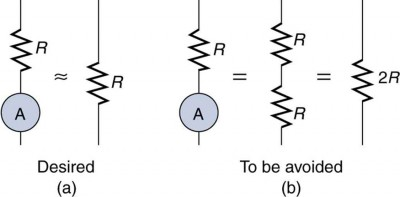Figure 7. (a) An ammeter normally has such a small resistance that the total series resistance in the branch being measured is not appreciably increased. The circuit is essentially unaltered compared with when the ammeter is absent. (b) Here the ammeter’s resistance is the same as that of the branch, so that the total resistance is doubled and the current is half what it is without the ammeter. This significant alteration of the circuit is to be avoided.

One solution to the problem of voltmeters and ammeters interfering with the circuits being measured is to use galvanometers with greater sensitivity. This allows construction of voltmeters with greater resistance and ammeters with smaller resistance than when less sensitive galvanometers are used. There are practical limits to galvanometer sensitivity, but it is possible to get analog meters that make measurements accurate to a few percent. Note that the inaccuracy comes from altering the circuit, not from a fault in the meter.

### Connections: Limits to Knowledge

Making a measurement alters the system being measured in a manner that produces uncertainty in the measurement. For macroscopic systems, such as the circuits discussed in this module, the alteration can usually be made negligibly small, but it cannot be eliminated entirely. For submicroscopic systems, such as atoms, nuclei, and smaller particles, measurement alters the system in a manner that cannot be made arbitrarily small. This actually limits knowledge of the system—even limiting what nature can know about itself. We shall see profound implications of this when the Heisenberg uncertainty principle is discussed in the modules on quantum mechanics.

There is another measurement technique based on drawing no current at all and, hence, not altering the circuit at all. These are called null measurements and are the topic of Null Measurements. Digital meters that employ solid-state electronics and null measurements can attain accuracies of one part in 106.

Digital meters are able to detect smaller currents than analog meters employing galvanometers. How does this explain their ability to measure voltage and current more accurately than analog meters?

#### Solution

Since digital meters require less current than analog meters, they alter the circuit less than analog meters. Their resistance as a voltmeter can be far greater than an analog meter, and their resistance as an ammeter can be far less than an analog meter. Consult Figure 2 and Figure 3 and their discussion in the text.

## PhET Explorations: Circuit Construction Kit (DC Only), Virtual Lab

Stimulate a neuron and monitor what happens. Pause, rewind, and move forward in time in order to observe the ions as they move across the neuron membrane.

## Section Summary

• Voltmeters measure voltage, and ammeters measure current.
• A voltmeter is placed in parallel with the voltage source to receive full voltage and must have a large resistance to limit its effect on the circuit.
• An ammeter is placed in series to get the full current flowing through a branch and must have a small resistance to limit its effect on the circuit.
• Both can be based on the combination of a resistor and a galvanometer, a device that gives an analog reading of current.
• Standard voltmeters and ammeters alter the circuit being measured and are thus limited in accuracy.

### Conceptual Questions

1. Why should you not connect an ammeter directly across a voltage source as shown in Figure 9? (Note that script E in the figure stands for emf.)

2. Suppose you are using a multimeter (one designed to measure a range of voltages, currents, and resistances) to measure current in a circuit and you inadvertently leave it in a voltmeter mode. What effect will the meter have on the circuit? What would happen if you were measuring voltage but accidentally put the meter in the ammeter mode?

3. Specify the points to which you could connect a voltmeter to measure the following potential differences in Figure 10: (a) the potential difference of the voltage source; (b) the potential difference across R1; (c) across R2; (d) across R3; (e) across R2 and R3. Note that there may be more than one answer to each part.

4. To measure currents in Figure 10, you would replace a wire between two points with an ammeter. Specify the points between which you would place an ammeter to measure the following: (a) the total current; (b) the current flowing through R1; (c) through R2; (d) through R3. Note that there may be more than one answer to each part.

### Problems & Exercises

1. What is the sensitivity of the galvanometer (that is, what current gives a full-scale deflection) inside a voltmeter that has a 1.00-MΩ resistance on its 30.0-V scale?

2. What is the sensitivity of the galvanometer (that is, what current gives a full-scale deflection) inside a voltmeter that has a 25.0-kΩ resistance on its 100-V scale?

3. Find the resistance that must be placed in series with a 25.0-Ω galvanometer having a 50.0-μA sensitivity (the same as the one discussed in the text) to allow it to be used as a voltmeter with a 0.100-V full-scale reading.

4. Find the resistance that must be placed in series with a 25.0-Ω galvanometer having a 50.0-μA sensitivity (the same as the one discussed in the text) to allow it to be used as a voltmeter with a 3000-V full-scale reading. Include a circuit diagram with your solution.

5. Find the resistance that must be placed in parallel with a 25.0-Ω galvanometer having a 50.0-μA sensitivity (the same as the one discussed in the text) to allow it to be used as an ammeter with a 10.0-A full-scale reading. Include a circuit diagram with your solution.
6. Find the resistance that must be placed in parallel with a 25.0-Ω galvanometer having a 50.0-μA sensitivity (the same as the one discussed in the text) to allow it to be used as an ammeter with a 300-mA full-scale reading.

7. Find the resistance that must be placed in series with a 10.0-Ω galvanometer having a 100-μA sensitivity to allow it to be used as a voltmeter with: (a) a 300-V full-scale reading, and (b) a 0.300-V full-scale reading.

8. Find the resistance that must be placed in parallel with a 10.0-Ω galvanometer having a 100-μA sensitivity to allow it to be used as an ammeter with: (a) a 20.0-A full-scale reading, and (b) a 100-mA full-scale reading.

9. Suppose you measure the terminal voltage of a 1.585-V alkaline cell having an internal resistance of 0.100Ω by placing a 1.00-kΩ voltmeter across its terminals. (See Figure 11.) (a) What current flows? (b) Find the terminal voltage. (c) To see how close the measured terminal voltage is to the emf, calculate their ratio.

10. Suppose you measure the terminal voltage of a 3.200-V lithium cell having an internal resistance of 5.00 Ω by placing a 1.00-kΩ voltmeter across its terminals. (a) What current flows? (b) Find the terminal voltage. (c) To see how close the measured terminal voltage is to the emf, calculate their ratio.

11. A certain ammeter has a resistance of 5.00 × 10−5Ω on its 3.00-A scale and contains a 10.0-Ω galvanometer. What is the sensitivity of the galvanometer?

12. A 1.00-MΩ voltmeter is placed in parallel with a 75.0-kΩ resistor in a circuit. (a) Draw a circuit diagram of the connection. (b) What is the resistance of the combination? (c) If the voltage across the combination is kept the same as it was across the 75.0-kΩ resistor alone, what is the percent increase in current? (d) If the current through the combination is kept the same as it was through the 75.0-kΩ resistor alone, what is the percentage decrease in voltage? (e) Are the changes found in parts (c) and (d) significant? Discuss.

13. A 0.0200-Ω ammeter is placed in series with a 10.00-Ω resistor in a circuit. (a) Draw a circuit diagram of the connection. (b) Calculate the resistance of the combination. (c) If the voltage is kept the same across the combination as it was through the 10.00-Ω resistor alone, what is the percent decrease in current? (d) If the current is kept the same through the combination as it was through the 10.00-Ω resistor alone, what is the percent increase in voltage? (e) Are the changes found in parts (c) and (d) significant? Discuss.

14. Unreasonable Results Suppose you have a40.0-Ω galvanometer with a 25.0-μA sensitivity. (a) What resistance would you put in series with it to allow it to be used as a voltmeter that has a full-scale deflection for 0.500 mV? (b) What is unreasonable about this result? (c) Which assumptions are responsible?

15. Unreasonable Results (a) What resistance would you put in parallel with a 40.0-Ω galvanometer having a 25.0-μA sensitivity to allow it to be used as an ammeter that has a full-scale deflection for 10.0-μA? (b) What is unreasonable about this result? (c) Which assumptions are responsible?

## Glossary

voltmeter:
an instrument that measures voltage
ammeter:
an instrument that measures current
analog meter:
a measuring instrument that gives a readout in the form of a needle movement over a marked gauge
digital meter:
a measuring instrument that gives a readout in a digital form
galvanometer:
an analog measuring device, denoted by G, that measures current flow using a needle deflection caused by a magnetic field force acting upon a current-carrying wire
current sensitivity:
the maximum current that a galvanometer can read
full-scale deflection:
the maximum deflection of a galvanometer needle, also known as current sensitivity; a galvanometer with a full-scale deflection of 50 μA has a maximum deflection of its needle when 50 μA flows through it
shunt resistance:
a small resistance R placed in parallel with a galvanometer G to produce an ammeter; the larger the current to be measured, the smaller R must be; most of the current flowing through the meter is shunted through R to protect the galvanometer

### Selected Solutions to Problems & Exercises

1. 30 μA

3. 1.98 kΩ

5. 1.25 × 10Ω

7. (a) 3.00 MΩ (b) 2.99 kΩ

9. (a) 1.58 mA (b) 1.5848 V (need four digits to see the difference) (c) 0.99990 (need five digits to see the difference from unity)

11. 15.0 μA

12.

(a)

(b) 10.02 Ω

(c) 0.9980, or a 2.0 × 10–1 percent decrease

(d) 1.002, or a 2.0 × 10–1 percent increase

(e) Not significant.

15. (a) −66.7 Ω (b) You can’t have negative resistance. (c) It is unreasonable that IG is greater than Itot (see Figure 5). You cannot achieve a full-scale deflection using a current less than the sensitivity of the galvanometer.